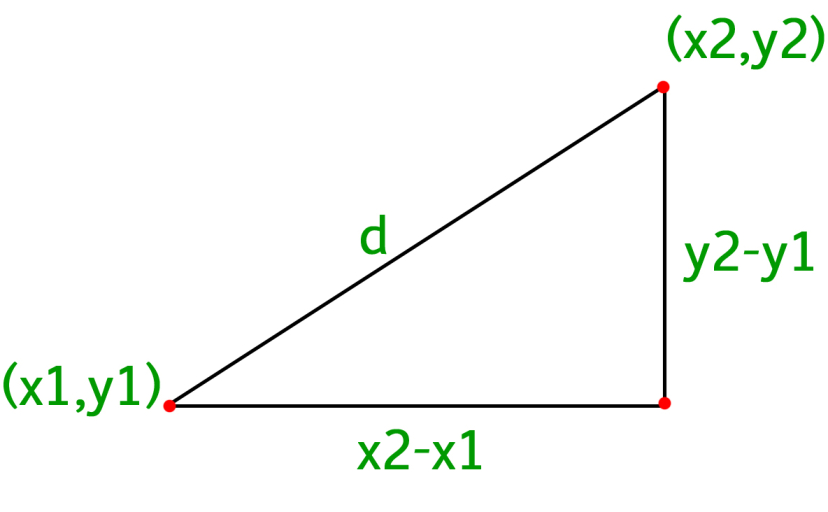Open in App
Not now

# C program to find the Euclidean distance between two points

• Last Updated : 04 Nov, 2021

Given four integers x1, y1, x2 and y2, which represents two coordinates (x1, y1) and (x2, y2) of a two-dimensional graph. The task is to find the Euclidean distance between these two points.

Euclidean distance between two points is the length of a straight line drawn between those two given points.

Examples:

Input: x1, y1 = (3, 4)
x2, y2 = (7, 7)
Output: 5

Input: x1, y1 = (3, 4)
x2, y2 = (4, 3)
Output: 1.41421

Approach: Since the Euclidean distance is nothing but the straight line distance between two given points, therefore the distance formula derived from the Pythagorean theorem can be used. The formula for distance between two points (x1, y1) and (x2, y2) isWe can get the above formula by simply applying the Pythagoras theoremBelow is the implementation of the above approach

## C

 // C program for the above approach  #include #include   // Function to calculate distancefloat distance(int x1, int y1, int x2, int y2){    // Calculating distance    return sqrt(pow(x2 - x1, 2)                + pow(y2 - y1, 2) * 1.0);}  // Driver Codeint main(){    printf("%.2f", distance(3, 4, 4, 3));    return 0;}

Output

1.41

Time Complexity: O(1)
Auxiliary Space: O(1)

My Personal Notes arrow_drop_up